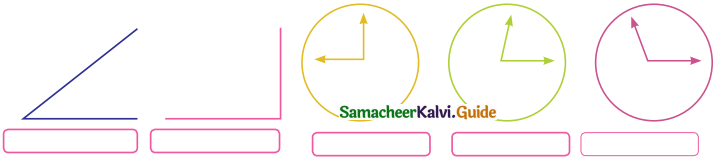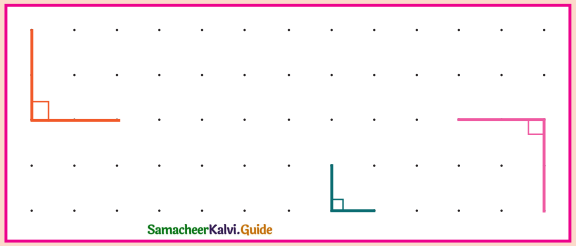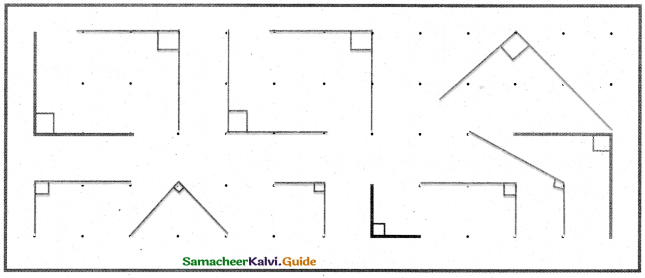Students can download 5th Maths Term 1 Chapter 1 Geometry InText Questions and Answers, Notes, Samacheer Kalvi 5th Maths Guide Pdf helps you to revise the complete Tamilnadu State Board New Syllabus, helps students complete homework assignments and to score high marks in board exams.

## Tamilnadu Samacheer Kalvi 5th Maths Solutions Term 1 Chapter 1 Geometry InText Questions

Acitivity (Text Book Page No. 4)

I. Write the 3-D shapes lying around us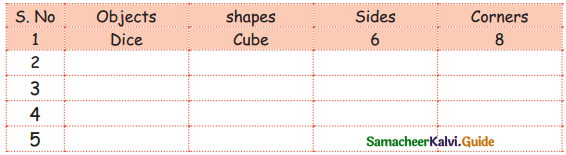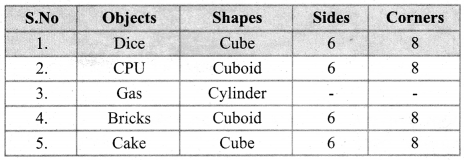II. What will you observe, if you look at this object from the front?

Question 1.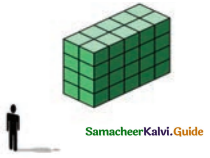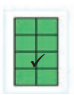Question 2.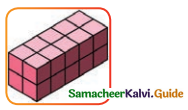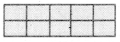III. What will you observe, if you look at this object from the sideways?

Question 1.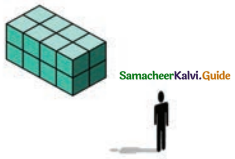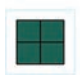Question 2.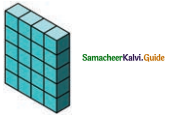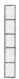Practice (Text Book Page No. 8)

Look at the following shapes. Draw that how will it be changed after 1/3 and 1/6 of a turn?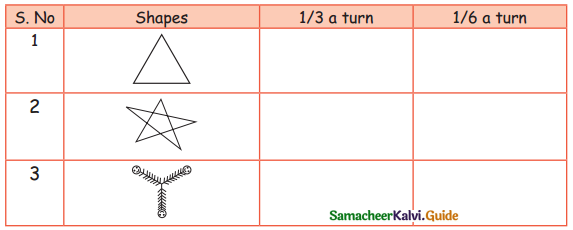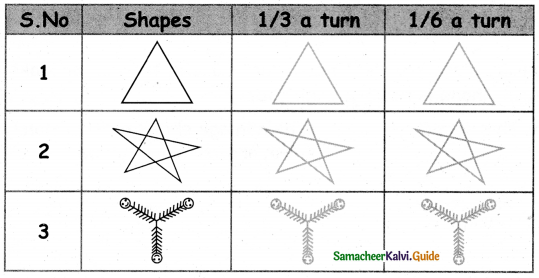Activity (Text Book Page No. 9)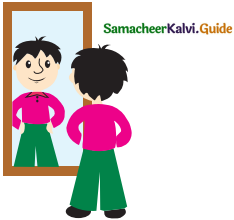While standing in front of a mirror, see your image.

Observe your image in the mirror when moving back and coming front to the mirror again, what do you infer?

Question 1.
Your image in the mirror is __________ (bigger, smaller, same size)
same size

Question 2.
When you go back, your image is moving _________ (backward, forward)
backward

Question 3.
The distance between you and mirror and the distance between you and your image is ________ . (equal, unequal)
equal

Question 4.
When you come forward to the mirror, your images is moving _________ . (forward, backward)
forward

Question 5.
When you raise your right hand, the image in the mirror looks like, _________ hand is raising. (right, left)
left

Question 6.
When you raise your left hand, the image in the mirror looks like, _________ hand is raising, (right, left)
right

Question 7.
Look at this shape: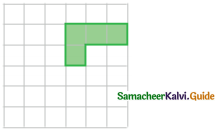Which image shows a reflection? ✓ the answer given below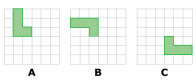BTry yourself (Text Book Page No. 10)

Draw some of your favourite shapes and draw its reflection images on a chart and show it to your teacher.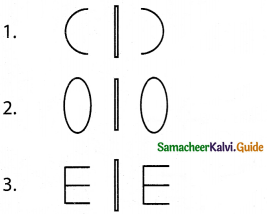Project (Text Book Page No. 12)

Question 1.
List out 2 symmetrical objects that you know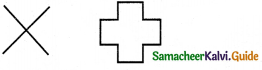Question 2.
Tick among the following picture, having symmetry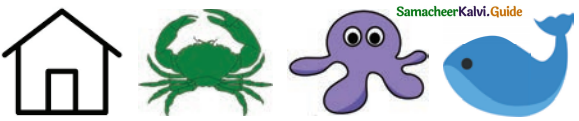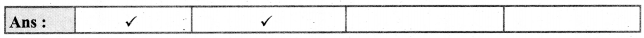Question 3.
Complete the other half to make the given figure as symmetrical.
Question 1.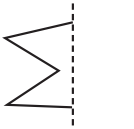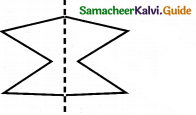Question 2.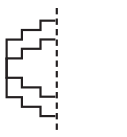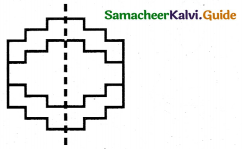Question 4.
Draw the lines of symmetry for the following figures then count and write the number of lines.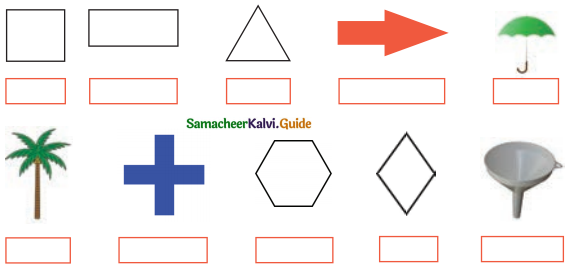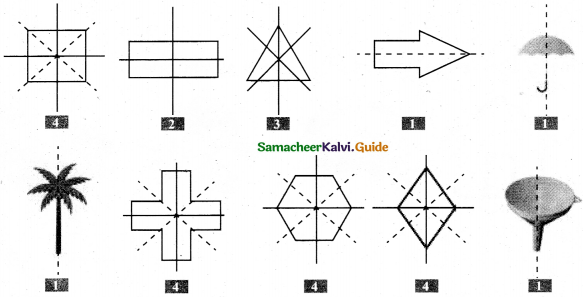Think it (Text Book page No. 13)

Question 1.
Can we divide the irregular solids symmetrically? if no why?
No, they are not equal parts.
Example: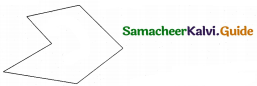Question 2.
Write the english alphabets that can’t be divided symmetrically?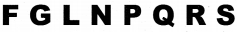Question 3.
Write the english alphabets which are divided symmetrically?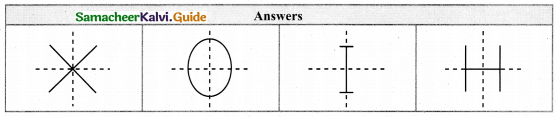Question 4.
Circle has many lines of symmetry. Is is true? why?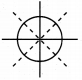It has infinite lines of symmetry. The circle is symmetric along all the diameters.

Question 5.
Find the three numbers between 1 and 9 that can be divided symmetrically?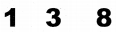Question 6.
Find two numbers between 1 to 9 having two lines of symmetry?
8, [1, 2, 3, 4, 5, 6, 7, and 9 are unsymmetrical 3 has a horizontal line of symmetry 8 has a vertical and a horizontal line of symmetry]Try These (Text Book Page No. 14)

Find out which of these can be made into a box by folding along the dotted lines. Put a tick mark for the correct option.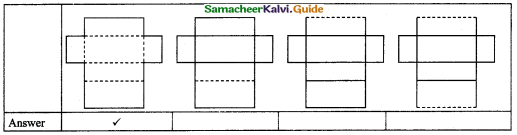Activity (Text Book Page No. 16)

Match the net with the shape you will get by folding.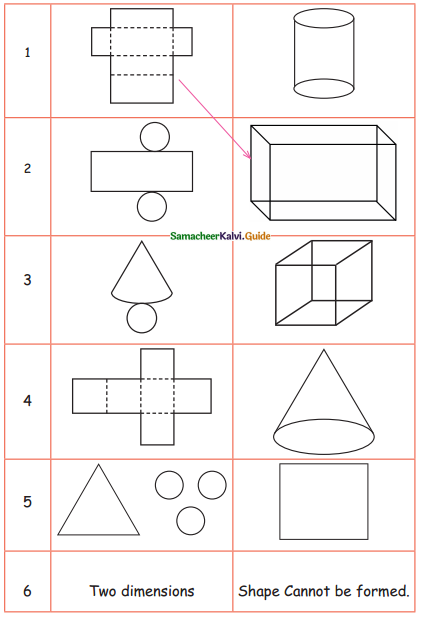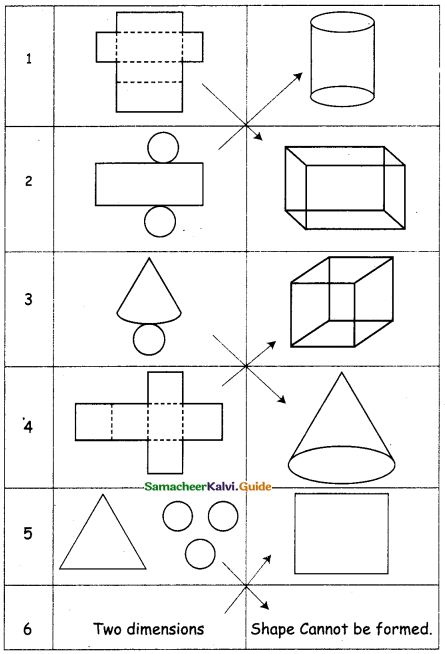Introduction of Angles (Text Book Page No. 18)

To get the feel of an angle through observation of objects and by paper folding: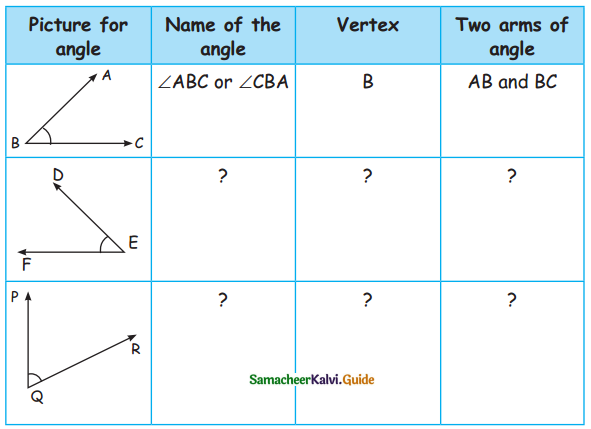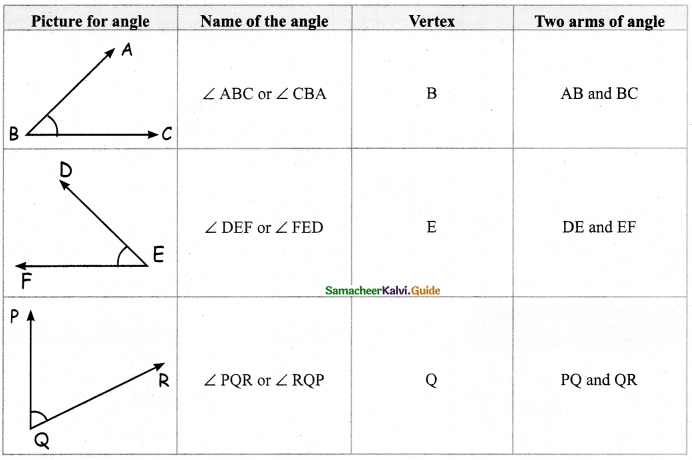Name the types of angles formed in the following items.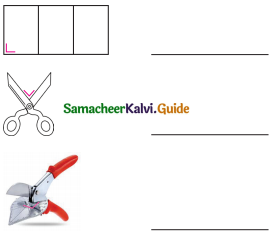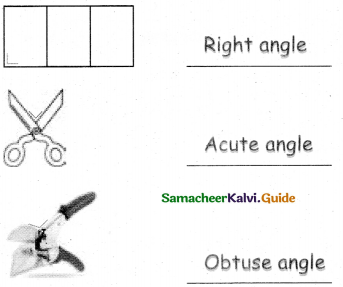Try These (Text Book Page No: 20)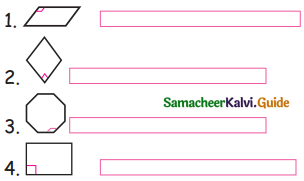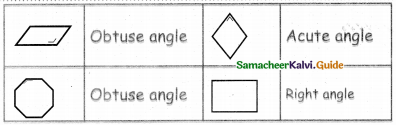Do yourself (Text Book Page No. 21)

Draw 5 objects with right angle.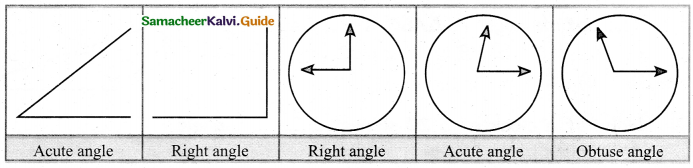Try These (Text Book Page No. 21)

Question 1.
Classify the following angles (acute angle, obtuse angle and right angle)
30°, 45°, 60°, 90°, 120°, 130°, 170°, 75°

 1. Acute angles 30°, 45°, 60°, 75° 2. Right angle 90° 3. Obtuse angle 120°, 130°, 170°

Question 2.
Observe the following pictures and write the name of the angles in their box.